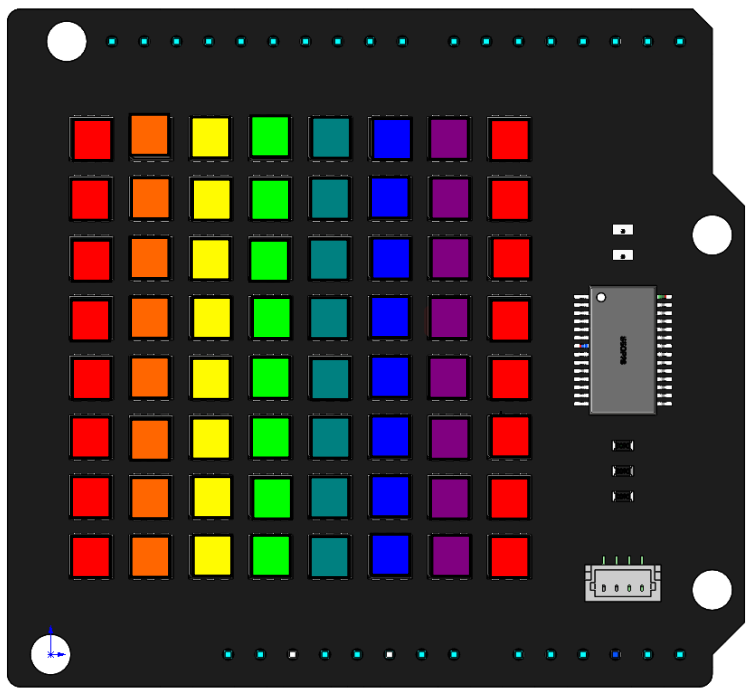# Dazzling Light¶

In the previous project, we learned to use some simple functions to make RGB Matrix Shield work. So here, we will use the `draw_line()` function with different colors to make RGB Matrix HAT make more cool effects.Code

We have written two light blinking modes, `dazzling_light()` and `dazzling_light()2` for reference. When the program is running, you will first see the RGB matrix shield flowing on displaying different colors. After a while, you will notice that the flow of light becomes more smooth.

How it works?

```for (int i=0; i<50; i++){
dazzling_light();
}
for (int i=0; i<5; i++){
dazzling_light2();
}
```

The main logic is to call the `dazzling_light()` function 50 times and then call the `dazzling_light2()` function 5 times.

```byte line = {{0, 0, 0, 7},
{1, 0, 1, 7},
{2, 0, 2, 7},
{3, 0, 3, 7},
{4, 0, 4, 7},
{5, 0, 5, 7},
{6, 0, 6, 7},
{7, 0, 7, 7}};
```

Define a two-dimensional array `line` to store the starting and ending coordinates of the 8 vertical lines.

```byte color = {{255,0,0},
{255,102,0},
{255,255,0},
{0,255,0},
{0,128,128},
{0,0,255},
{128,0,128}};
```

Define a two-dimensional array `color` to store the 7 colors, red, orange, yellow, green, blue, blue and purple.

```  int i = 0;
void dazzling_light(){
for (int j=0; j<8; j++){
draw_line(line[j],color[i],color[i],color[i]);
i++;
if (i == 6){
i = 0;
}
}
image();
}
```

The `dazzling_light()` function is to write different colors (red, orange, yellow, green, blue, blue and purple) to the 8 vertical lines, where the first and last lines are red.

The `for` loop traverses the array `line[]` and draws eight vertical lines on the RGB matrix shield with `draw_line()`. The colors are chosen from the array `color`, for example, `color` represents the first element {255, 0, 0}, while `color` represents 255.

```void dazzling_light2(){
for (long firstPixelHue = 0; firstPixelHue < 65536; firstPixelHue += 500) {
for (int j=0; j<8; j++){
long pixelHue = firstPixelHue + (j * 65536L / 16);
draw_line(line[j], gamma32(ColorHSV(pixelHue)));
}
image();
}
}
```

When you call the `dazzling_light2()` function, you will notice a softer flow of colors. This is because we have split the colors into more colors, making the transition between colors more smooth.

Two for loops are defined in `dazzling_light2()`. The inner loop is to fill the eight vertical lines with eight colors, and the outer loop is to add a value to each color to switch to the next color to achieve the effect of color flow.

Here `ColorHSV()` and `gamma32()` are functions packaged in the library. The former is used to handle decimal numbers, which is equivalent to mapping all the colors of the RGB matrix shield to the range 0 to 65536. `gamma32()` is used for transcoding, converting the return value of `ColorHSV()` into an acceptable argument to `draw_line()`.